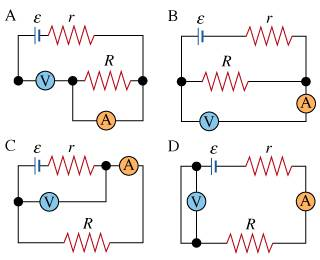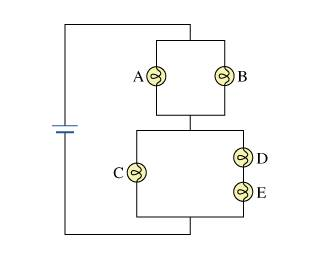# Diagrams of Ammeters

## Homework Statement

In which diagram(s) (labeled A - D) does the ammeter correctly measure the current through the battery?## Homework Equations

I don't believe there are any here, just to know that the ammeter is in series with the current that is passing through it and is measuring that current.

## The Attempt at a Solution

The thing is I'm not sure where the current is coming out of, would I be correct in saying the emf is the source of the current throughout the whole of the battery? If that was the case I was thinking the only current that the ammeter is in series with with battery C but that answer was incorrect (masteringphysics), or would it be that the ammeter is in series with the potential difference measured by the voltmeters?

any help is greatly appreciated.

Chi Meson
Homework Helper
There is a correct answer here, and it's obvious. THink of the current as a continuously flowing loop of water through closed pipes, and the battery is the pump that moves the water. Remember that no current flows through the voltmeter.

Note: only two of these four circuits demonstrate correct placement of both meters. In one of them, one meter is misplaced; in another, both are misplaced.

Remember that the voltmeters that they give are probably "ideal" meaning they have infinite resistance. From this, there are two that you can throw away, I believe.

Redbelly98
Staff Emeritus
Homework Helper
The question is, for which diagram is the ammeter correct?

If we assume an ideal (infinite resistance) voltmeter, there are two correct answers. This is probably not what the problem author had in mind, so I conclude that the voltmeter is non-ideal, and can draw some current.

If the voltmeter can draw current, there is just one correct answer for a correct ammeter reading.

The question is, for which diagram is the ammeter correct?

If we assume an ideal (infinite resistance) voltmeter, there are two correct answers. This is probably not what the problem author had in mind, so I conclude that the voltmeter is non-ideal, and can draw some current.

If the voltmeter can draw current, there is just one correct answer for a correct ammeter reading.

no it is an ideal voltmeter so the answer is ammeters C & D, thanks for the help on this one guys, but unfortunately I do have another one that's a little harder:I am trying to find the order of brightness for the lightbulbs; I know that it will depend on the fraction of voltage divided among the lightbulbs and I know the voltage is divided across the each bulb depending on the resistance encountered in each pathway (with D and E in series with eachother), but from there I'm not sure how to set up a fraction for the division of voltage, any help is greatly appreciated.

Redbelly98
Staff Emeritus
Homework Helper
no it is an ideal voltmeter so the answer is ammeters C & D

I reread the problem statement, and see now that they are allowing for more than one answer. Thanks for clarifying.

New problem:
I am trying to find the order of brightness for the lightbulbs; I know that it will depend on the fraction of voltage divided among the lightbulbs and I know the voltage is divided across the each bulb depending on the resistance encountered in each pathway (with D and E in series with eachother), but from there I'm not sure how to set up a fraction for the division of voltage, any help is greatly appreciated.

Let each lightbulb have a resistance of R. How is the voltage divided between the upper pair vs. the bottom three bulbs?

I reread the problem statement, and see now that they are allowing for more than one answer. Thanks for clarifying.

New problem:

Let each lightbulb have a resistance of R. How is the voltage divided between the upper pair vs. the bottom three bulbs?

just looking at the diagram I would say 2/3 but I'm not sure that's correct, considering D and E are in series with eachother.

Redbelly98
Staff Emeritus
Homework Helper
No. Use the rules for combining resistors in parallel & series.

What is the net resistance of the upper pair?
What is the net resistance of the lower 3-bulb combination?

No. Use the rules for combining resistors in parallel & series.

What is the net resistance of the upper pair?
What is the net resistance of the lower 3-bulb combination?

well for D and E Rtot1 = Rd+Re

for the others that are in parallel, 1/Rtot = 1/Ra+1/Rb or I/Vab = 1/Ra+1/Rb

and C is in parallel with Rtot1 so 1/Rtot2 = 1/Rtot1+1/Rc or I/Vtot2 = 1/Rtot1+1/Rc

so Rtot for a and b is Ra+Rb/RaRb

and Rtot2 = Rtot1Rc/Rtot1Rc

so these would add up and give me the total resistance on each bulb?

okay, I added up all the resistances and got this:
3/2R (the resistance of the 3 bulb combo) and 2/R (the upper pair) = 7/(2R) as the total resistance is this correct?
also, since I need to find the current through bulb c, so I'm thinking I just need the resistance of the 3bulb combo and they're potential difference between the bulb c and the bulbs d and e.

Last edited:
Redbelly98
Staff Emeritus